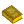## Complex Analysis

The study of Complex Numbers, their Derivatives, manipulation, and other properties. Complex analysis is an extremely powerful tool with an unexpectedly large number of practical applications to the solution of physical problems. Contour Integration, for example, provides a method of computing difficult Integrals by investigating the singularities of the function in regions of the Complex Plane near and between the limits of integration.

The most fundamental result of complex analysis is the Cauchy-Riemann Equations, which give the conditions a Function must satisfy in order for a complex generalization of the Derivative, the so-called Complex Derivative, to exist. When the Complex Derivative is defined ``everywhere,'' the function is said to be Analytic. A single example of the unexpected power of complex analysis is Picard's Theorem, which states that an Analytic Function assumes every Complex Number, with possibly one exception, infinitely often in any Neighborhood of an Essential Singularity!

See also Analytic Continuation, Branch Cut, Branch Point, Cauchy Integral Formula, Cauchy Integral Theorem, Cauchy Principal Value, Cauchy-Riemann Equations, Complex Number, Conformal Map, Contour Integration, de Moivre's Identity, Euler Formula, Inside-Outside Theorem, Jordan's Lemma, Laurent Series, Liouville's Conformality Theorem, Monogenic Function, Morera's Theorem, Permanence of Algebraic Form, Picard's Theorem, Pole, Polygenic Function, Residue (Complex Analysis)

ReferencesComplex Analysis

Arfken, G. ``Functions of a Complex Variable I: Analytic Properties, Mapping'' and ``Functions of a Complex Variable II: Calculus of Residues.'' Chs. 6-7 in Mathematical Methods for Physicists, 3rd ed. Orlando, FL: Academic Press, pp. 352-395 and 396-436, 1985.

Boas, R. P. Invitation to Complex Analysis. New York: Random House, 1987.

Churchill, R. V. and Brown, J. W. Complex Variables and Applications, 6th ed. New York: McGraw-Hill, 1995.

Conway, J. B. Functions of One Complex Variable, 2nd ed. New York: Springer-Verlag, 1995.

Forsyth, A. R. Theory of Functions of a Complex Variable, 3rd ed. Cambridge, England: Cambridge University Press, 1918.

Lang, S. Complex Analysis, 3rd ed. New York: Springer-Verlag, 1993.

Morse, P. M. and Feshbach, H. ``Functions of a Complex Variable'' and ``Tabulation of Properties of Functions of Complex Variables.'' Ch. 4 in Methods of Theoretical Physics, Part I. New York: McGraw-Hill, pp. 348-491 and 480-485, 1953.